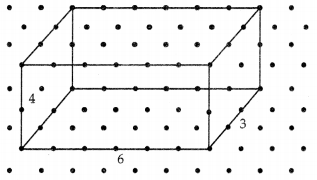# DAV Class 7 Maths Chapter 14 Worksheet 4 Solutions

The DAV Class 7 Maths Book Solutions and DAV Class 7 Maths Chapter 14 Worksheet 4 Solutions of Visualising Solids offer comprehensive answers to textbook questions.

## DAV Class 7 Maths Ch 14 WS 4 Solutions

Question 1.
Draw an Solution:
Here, we sketch a cuboid with dimensions 6 × 3 × 4 which means that the length, breadth and height are 6, 3, and 4 respectively. The distance between two dots is taken as one unit.